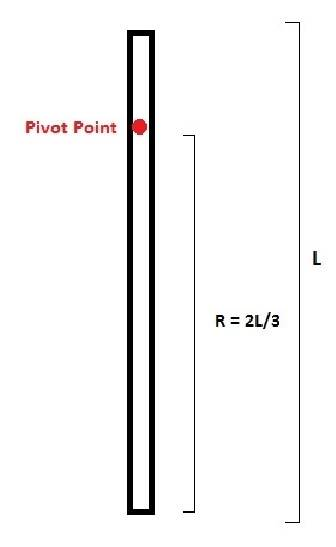# How can I find the inertia of a rod with a pivot point?

I have a question on finding the inertia of a rod with a pivot point somewhere along it's length.

So the inertia of a rod is IStick cm = 1/12 *m*L2
Does L2 = (2L/3)2 = 4L2/9 -> 1/12 *m*[4L2/9] or is it something like
m*L2/12 + m* (2L/3)2?

If it's the second one then may I ask why it's that?

PictureRevise parallel axis theorem; I = Ic + (md^2) Ic is MI about CM and d is the distance between parallel axes passing through CM and pivot. It can be proved first and then can be used.

Revise parallel axis theorem; I = Ic + (md^2) Ic is MI about CM and d is the distance between parallel axes passing through CM and pivot. It can be proved first and then can be used.

so m*L2/12 + m* (2L/3)2
where Ic = m*L2/12
and md^2 = m* (2L/3)2

when you said "MI about CM" you mean the mass around the center mass which is the entire rod? As for d is the "distance between parallel axes passing through CM and pivot" meaning the length between the end of the stick pass the center mass where it ends at the pivot?

center of mass is at the mid point of rod at L/2 from one end and if pivot is at 2L/3 from one end then d = (2L/3) - (L/2) = L/6. Ic is the MI about the axis passing through the point at L/2 from one end.